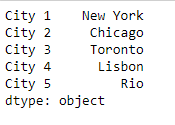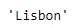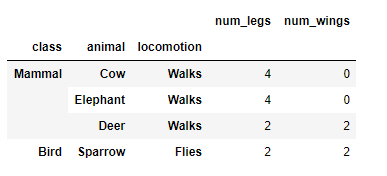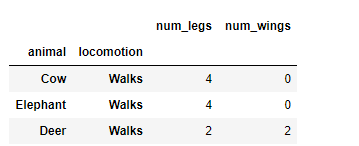Related Articles

# Python | Pandas Series.xs

• Last Updated : 28 Jan, 2019

Python is a great language for doing data analysis, primarily because of the fantastic ecosystem of data-centric python packages. Pandas is one of those packages and makes importing and analyzing data much easier.

Pandas series is a One-dimensional ndarray with axis labels. The labels need not be unique but must be a hashable type. The object supports both integer- and label-based indexing and provides a host of methods for performing operations involving the index.

Pandas` Series.xs()` function return a cross-section from the Series/DataFrame for the given key value.

Syntax:Series.xs(key, axis=0, level=None, drop_level=True)

Parameters :
key : Label contained in the index, or partially in a MultiIndex.
axis : Axis to retrieve cross-section on.
level : In case of a key partially contained in a MultiIndex, indicate which levels are used. Levels can be referred by label or position.
drop_level : If False, returns object with same levels as self.

Returns : Series or DataFrame

Example #1: Use `Series.xs()` function to return a cross-section of the given Series object for the passed key value.

 `# importing pandas as pd``import` `pandas as pd`` ` `# Creating the Series``sr ``=` `pd.Series([``'New York'``, ``'Chicago'``, ``'Toronto'``, ``'Lisbon'``, ``'Rio'``])`` ` `# Creating the row axis labels``sr.index ``=` `[``'City 1'``, ``'City 2'``, ``'City 3'``, ``'City 4'``, ``'City 5'``] `` ` `# Print the series``print``(sr)`

Output :Now we will use `Series.xs()` function to return the cross-section for the given series object.

 `# return cross-section corresponding to``# the 'City 4' label``sr.xs(key ``=` `'City 4'``)`

Output :As we can see in the output, the `Series.xs()` function has returned ‘Lisbon’ as the cross-section for the given Series object.

Example #2 : Use `Dataframe.xs()` function to return a cross-section of the given Dataframe object for the passed key value.

 `# importing pandas as pd``import` `pandas as pd`` ` `# Creating the Dataframe``df ``=` `pd.DataFrame({``'num_legs'``: [``4``, ``4``, ``2``, ``2``],``                  ``'num_wings'``: [``0``, ``0``, ``2``, ``2``],``                  ``'class'``: [``'Mammal'``, ``'Mammal'``, ``'Mammal'``, ``'Bird'``],``                  ``'animal'``: [``'Cow'``, ``'Elephant'``, ``'Deer'``, ``'Sparrow'``],``                  ``'locomotion'``: [``'Walks'``, ``'Walks'``, ``'Walks'``, ``'Flies'``]})`` ` `# setting the index``df ``=` `df.set_index([``'class'``, ``'animal'``, ``'locomotion'``])`` ` `# Print the Dataframe``print``(df)`

Output :Now we will use `Dataframe.xs()` function to return the cross-section for the given Dataframe object.

 `# return cross-section corresponding to``# the 'Mammal' label``sr.xs(key ``=` `'Mammal'``)`

Output :As we can see in the output, the `Dataframe.xs()` function has returned the cross-section of the given Dataframe object for the passed key value.

Attention geek! Strengthen your foundations with the Python Programming Foundation Course and learn the basics.

To begin with, your interview preparations Enhance your Data Structures concepts with the Python DS Course. And to begin with your Machine Learning Journey, join the Machine Learning – Basic Level Course

My Personal Notes arrow_drop_up